GRE Subject Test: Physics : Circular and Rotational Motion

Example Questions

Example Question #1 : Circular And Rotational Motion

What relation must be true between the minimum potential energy and the total energy of the satellite for the shape of its orbit to be circular?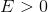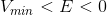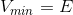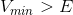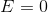Explanation:

For a gravitation potential, the total energy is: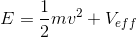, where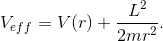When the energy equals the minimum potential energy, the satellite has just enough energy to maintain a circular orbit around the planet. When the potential energy is larger than the total energy, the satellite spirals in toward the planet. When the total energy is greater than zero, the satellite is deflected out into space.

All GRE Subject Test: Physics Resources